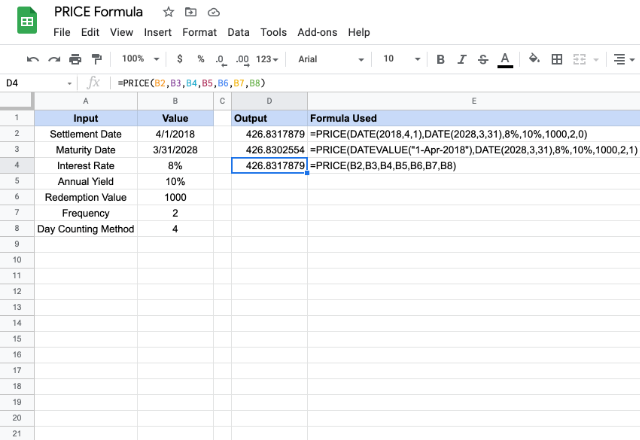# How to use the PRICE formula in Google SheetsGiven the periodic interest rate and expected yield, do you need to calculate the price of an investment or a security? The aptly named PRICE formula in Google Sheets helps us with that. To be able to use the formula, however, we will need additional information corresponding to the security, as explained below.

### Syntax

PRICE(settlement, maturity, rate, yield, redemption, frequency, [day_count_convention])

• settlement – the date when it is actually delivered to the buyer after issuing the security.
• maturity – the maturity date of the security, when the buyer can redeem it at face value.
• rate – the annualized interest rate at which the investment appreciates.
• yield – the annual yield that the buyer expects for the security.
• redemption  the redemption value of the security.
• frequency – the number of interest payments that buyer can pay per year (1, 2 or 4).
• day_count_convention – [OPTIONAL – 0 by default] – an indicator of the day count method that Google Sheets should consider. There are five different possible values for this parameter.
• 0 – assumes that there are 30 day months and 360 day years (US (NASD) 30/360). Ensures there are specific adjustments to the entered dates that usually are at the end of months.
• 1 – calculates based on the actual number of days between the specified dates, and the actual number of days in the intervening years.
• 2 – calculates based on the actual number of days between the specified dates, but assumes a 360 day year.
• 3 – evaluates the PRICE formula in Google Sheets based on the actual number of days between the specified dates, but assumes a 365 day year.
• 4 – very similar to the first option 0, except, it adjusts end-of-month dates according to European financial conventions.

### Usage: PRICE formula in Google Sheets

The formula might look a little complicated, but a few examples can establish a clear understanding for us. So let’s get digging straight into them. Please have a look at the screenshot below.We need to ensure the settlement and maturity parameters get valid dates as inputs. They can either references to other date type cells or results returned from formulas such as DATETO_DATE. Otherwise, the formula might return parsing errors.

The other parameters – rateyield, redemptionfrequency, and day_count_convention – can be direct numeric values or can be references to the cells holding the appropriate values. This is demonstrated in the examples above.

In all of the examples, we notice that the initial six parameters are essentially the same. Yet, the output values vary across the examples. This is because of the method with which the days are counted which we indicated using the day_count_convention parameter.

### PRICE formula

And there you go! Use the PRICE formula in Google Sheets to calculate the price of an investment.

If you’d like to learn more about the various formulas of Google Sheets, why not take a look at our blog posts on the SLN formula in Google Sheets .

Alternatively, check out related blog posts below!